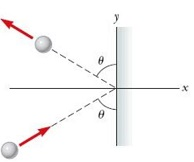# A 2.52-kg steel ball strikes a massive wall at 10.0 m/s at an angle of theta = 60.0 degree with...

## Question:

A 2.52-kg steel ball strikes a massive wall at 10.0 m/s at an angle of {eq}\theta \ = 60^\circ {/eq} with the plane of the wall. It bounces off the wall with the same speed and angle (see the figure below). If the ball is in contact with the wall for 0.194 s, what is the average force exerted by the wall on the ball?## Newton's Second Law of Motion:

When an external force is applied to an object, it causes a change in linear momentum of the object. According to Newton's second law of motion, the rate of change of linear momentum is equal to the external applied force. Mathematically:

• {eq}F_{ext} \ = \dfrac{\Delta p}{\Delta t} {/eq}

Where;

• {eq}F_{ext} {/eq} is the applied force
• {eq}p {/eq} is the linear momentum
• {eq}t{/eq} is the time

Given:

• Mass of the ball {eq}m = 2.52\ kg {/eq}
• Initial velocity of the ball, {eq}\vec u\ = 10 \sin 60^\circ \hat i \ + 10 \cos 60^\circ \hat...

Become a Study.com member to unlock this answer! Create your account